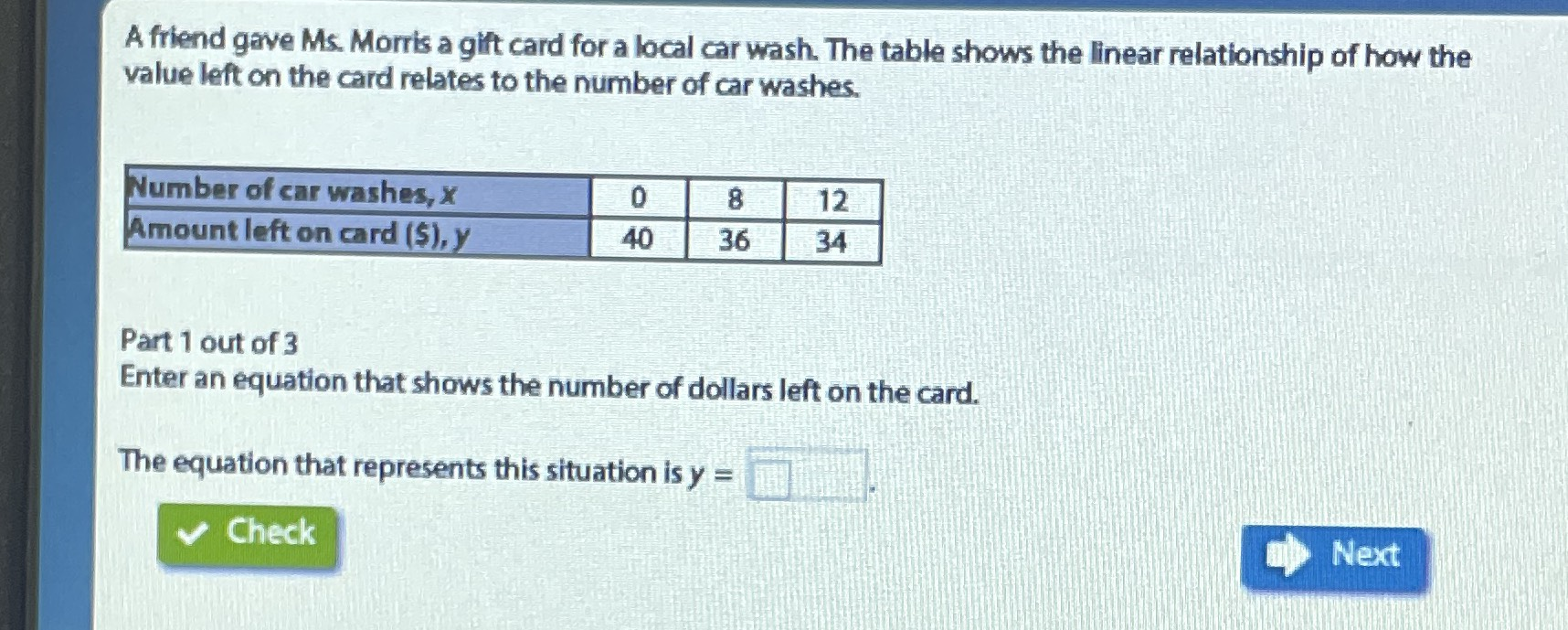### ¿Todavía tienes preguntas de matemáticas?

Pregunte a nuestros tutores expertos
Algebra
PreguntaA friend gave Ms. Morris a git card for a local car wash. The table shows the linear relationship of how the value left on the card relates to the number of car washes.

Part $$1$$ out of $$3$$

Enter an equation that shows the number of dollars left on the card.

The equation that represents this situation is $$y =$$

$$y= -\frac{1}{2} x+ 40$$